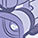Level 4
Level 4Hello,

It seems that the program of "range-angle-map.py" includes the element of DBM.

However, "range-angle-map.py" does not display 3D range-angle heat map.

Could you tell me why the element of DBM is included in "range-angle-map.py"?

Best regards, Shoko

1 SolutionModeratorModeratorHey @shoko_s

In rx_mask, Each antenna is assigned to a separate bit starting from LSB.

For example,

• rx-mask=5: 5=101, so RX1 and RX3 are activated
• rx-mask=7: 7=111, so RX1,RX2 and RX3 are activated
• rx-mask=1: 1=001, so RX1 is activated

Hope this helps, please go through the RDK documentation ->Data Structures to know functionalities of all ifxAvian functions.

Thankyou, Siddharth

10 RepliesModeratorModeratorHi @shoko_s

Before answering, am assuming you meant to say DBF( Digital beam formation) and not DBM.

So, The range angle map is a derived properties of the DBF algorithm, so the range angle map uses some components of the DBF to process the range angle map. So the DBF.py is included in range-angle-map.py

Please refer the RDK documentation for better understanding how DBF works.

Thankyou

SiddharthLevel 4
Level 4Hello Siddharth,

Thank you for your response. I'm sorry, It was a typing mistake.

I understand that why the element of DBF is included in "range-angle-map.py".

By the way, I have some questions about the program of  "DBF.py".

1) Please let me know why nothing is defined for num_antennas.

2) Please let me know why 27 is defined for num_beams.

Regards, ShokoModeratorModeratorHi @shoko_s

1) In the "range-angle-map.py", The num_antennas is taking the value from num_rx_antenna  when the dbf object is created.

num_rx_antenna  gets it's value from config  parameters

Look at this line for understanding- num_rx_antennas = num_rx_antennas_from_config(config)

The flow goes this way:

config.rx_mask ➡️ num_rx_antennas_from_config(config) ➡️ num_rx_antennas ➡️ num_antennas

2) num_beams equaling 27 is just a user defined value, you can try out any values for it. It just defines how many beams the FOV should be broken down into.

BR, SiddharthLevel 4
Level 4Hello Siddharth,

About 1), I don't understand how number of antenna related to range-angle-mapping on the program.

Doesn't this program perform AngleFFT (further FFT to the Doppler FFT result of each antenna)?

Regard, ShokoModeratorModeratorHi @shoko_s

• DBF is an algorithm which the other files like range-angle-map.py uses, DBF alone doesn't have any output. DBF is included in range-angle-map.py, where the value from rx_mask is derived by the DBF to calculate the number of antennas.
• The range-angle-map.py code file is implicitly linking to DBF.py using the command 'from DBF import DBF' and 'dbf = DBF(num_rx_antennas, num_beams = num_beams, max_angle_degrees = max_angle_degrees)' would ensure that the value configured for the num_rx_antennas is linked to both.

Hopes this helps you, Do post a reply if you have question or if you find a solution.
Thankyou

SiddharthLevel 4
Level 4Hello Siddharth,

Thanks for reply. Yes I already understand that DBF is an algorithm which the other files, and DBF alone doesn't have any output.

In the first place, I'd like to know- why nothing is defined for "num_antennas" in DBF.py, not "num_rx_antennas" in range-angle-map.py.

In the end... how many is the number of (virtual) RX antennas in DBF.py specifically?

Thank you, ShokoModeratorModeratorHi @shoko_s

"num_antennas" and "num_rx_antennas" are the same.  When the object is created, value from  "num_rx_antennas" is copied to "num_antennas".  In range-angle-map.py the variable is named "num_rx_antennas" and in DBF.py the variable is named "num_antennas" but they both have the same value.

Hope this makes it clear.

SiddharthLevel 4
Level 4Hello Siddharth,

Thank you for reply. I understand.ModeratorModeratorHey @shoko_s

In rx_mask, Each antenna is assigned to a separate bit starting from LSB.

For example,

• rx-mask=5: 5=101, so RX1 and RX3 are activated
• rx-mask=7: 7=111, so RX1,RX2 and RX3 are activated
• rx-mask=1: 1=001, so RX1 is activated

Hope this helps, please go through the RDK documentation ->Data Structures to know functionalities of all ifxAvian functions.

Thankyou, SiddharthLevel 4
Level 4